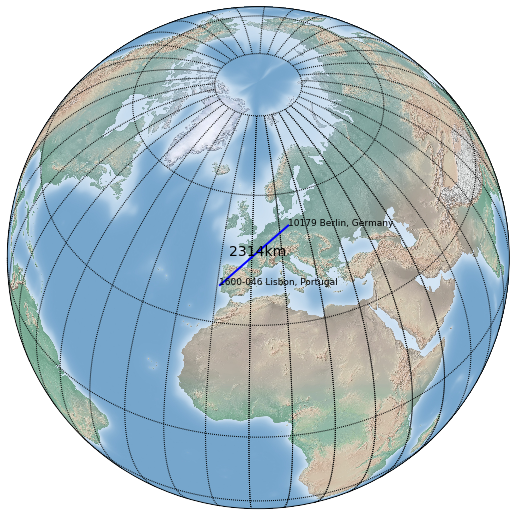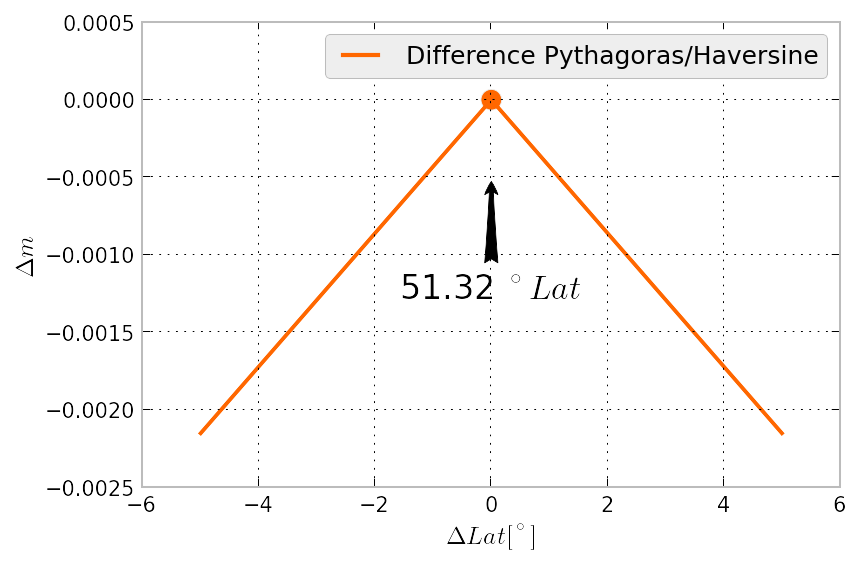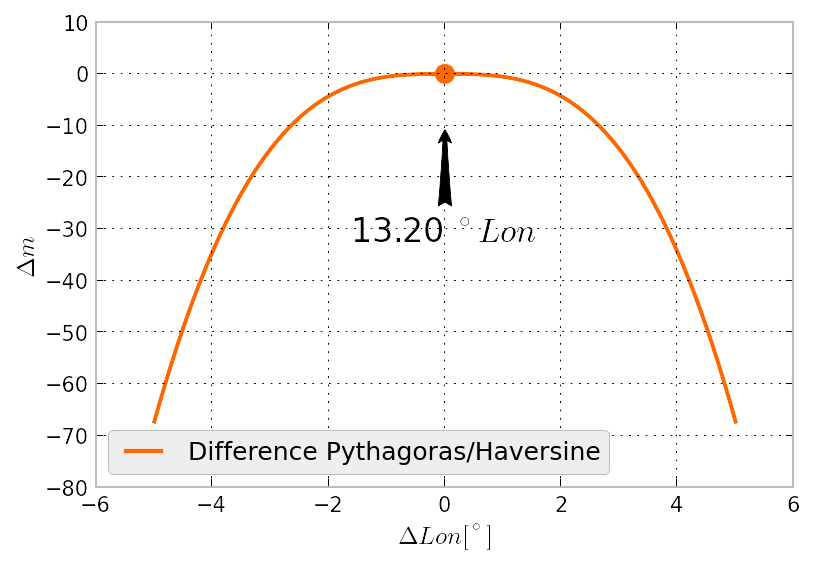# Latlon2meter

Python Script to calculate the Distance between two Lat/Lon Points

This project is maintained by balzer82

# LatLon2Meter

Python Script to calculate the distance between two points from Lat/Lon Coordinates

## How to use?

```python LatLon2Meter.py 38.7436265 -9.1602036 52.5075419 13.4261418
```

is doin this for you:

``````Calculating Distance from 1600-046 Lisbon, Portugal to 10179 Berlin, Germany
Entfernung aus Pythagoras:          2331854.029m (ca. 2331km)
Entfernung aus Seitencosinus:       2314672.226m (ca. 2314km)
Berechnung über Pythagoras macht -17.18km (0.74%) Fehler.
'Distance.png' saved. Done.
``````The coordinates (Lat/Lon) have to be in Decimal Degrees, not in DD°mm'ssss''

## What does this do?

Calculates the distance between two points with three different methods:

• Pythagoras
• Great Circle via Cosine (better)
• Haversine Formula

## Dependencies

• Matplotlib Basemap for Globe output
• requests for Reverse-Geoencoding of the Lat/Lon Coordinates to Adress

# LatLon2Meter Error